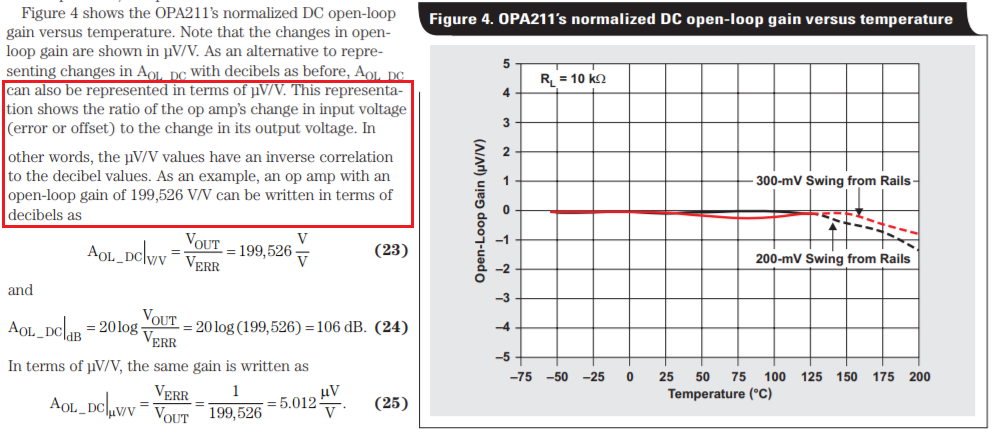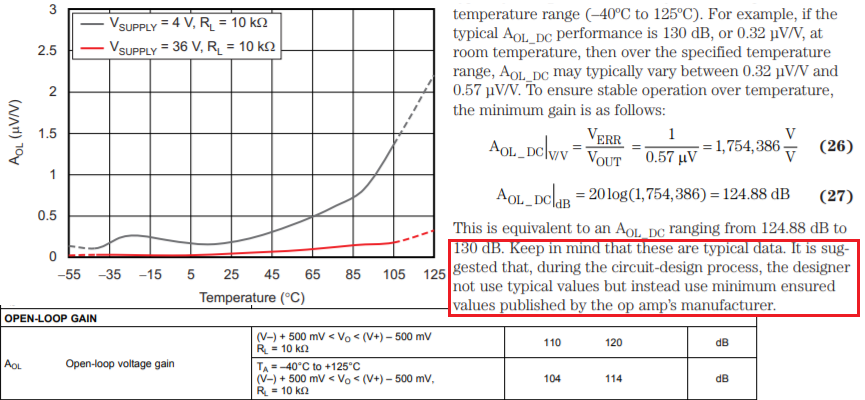If you have a related question, please click the "Ask a related question" button in the top right corner. The newly created question will be automatically linked to this question.

# OPA2180: open-loop gain vs temperature

Part Number: OPA2180
Other Parts Discussed in Thread: OPA180, OPA4180,

Hi Team,

Good day,

We received a query from customer and they want to understand more about the open-loop gain vs temperature of the said device. For me to not miss any information, I will copy the query below.

"I am trying to understand what datasheet is saying in regards to open loop gain.
Refer datasheet: OPA180, OPA2180, OPA4180, SBOS584E –NOVEMBER 2011–REVISED JUNE 2018, Figure 8, Open-Lop Gain vs Temperature.

Firstly, does the Vertical axis have the correct units? Marked as "Aol (uV / V)", which seems incorrect.
I suspect the units should be "Volts per micro-Volt", ie: "V / uV", ie: 1million.
Is there any reason that the usual units of dB are not used here?

OK, now assuming the vertical axis should be marked "uV / V", then a higher value means higher open-loop gain. Looking at the case when Temperature = 25C, the trace (line) coloured grey (Vsupply=4V, RL = 10kohm) has higher gain than the red trace (Vsupply=36V, RL = 10kohm). It is really the case that open-loop gain is higher when supply voltage is lower? The difference is not negligible, according to the chart the different is a factor of 5 or 10, and gets wider as temperature increases."

Thank you and looking forward for your kind response.

Regards,

Maynard

• Hello Maynard,

Firstly, does the Vertical axis have the correct units? Marked as "Aol (uV / V)", which seems incorrect.
I suspect the units should be "Volts per micro-Volt", ie: "V / uV", ie: 1million.
Is there any reason that the usual units of dB are not used here?

The reason the OPA2180 vertical open-loop gain (Aol) axis units are uV/V its is easier to see the small amount input change resulting in a one volt output change. Since the Aol is extremely high the input change is only a fraction of a microvolt for that output change. This very expanded scale shows how the OPA2180 input voltage changes with increased temperature indicating that the Aol is decreasing.

OK, now assuming the vertical axis should be marked "uV / V", then a higher value means higher open-loop gain. Looking at the case when Temperature = 25C, the trace (line) coloured grey (Vsupply=4V, RL = 10kohm) has higher gain than the red trace (Vsupply=36V, RL = 10kohm). It is really the case that open-loop gain is higher when supply voltage is lower? The difference is not negligible, according to the chart the different is a factor of 5 or 10, and gets wider as temperature increases."

Yes. The OPA2180 open-loop gain is higher with higher supply voltage. This occurs because the internal transistors have higher voltage across their collector to emitter witht he higher supply voltage. The transistors are operate in the flatter, active region of the characters curve where the rc'e is higher. Therefore, the developed higher voltage gain.

The information presented in Figure 8, Open-Loop Gain vs Temperature, is based on measured data so it is representative of the OPA2180 performance.

Regards, Thomas

Precision Amplifiers Applications Engineering

• Hi Maynard,

Q: Is there any reason that the usual units of dB are not used here?

Open-loop gain vs. temperature presented in uV/V is normalized, which is an alternative way to present changes in Aol. It has the same meaning  as dB technically, except the output/input ratio is inverted and presented as uV/V.  Also, the see the attached link below.Q: It is really the case that open-loop gain is higher when supply voltage is lower? The difference is not negligible, according to the chart the different is a factor of 5 or 10, and gets wider as temperature increases."

The part of Open-Loop Gain differences are contributed by Verr/Vout, since 4V is significantly smaller than 36V in supply voltage, the errors in ratios will be relative larger. In a design, you normally do not use the op amp in open loop configuration. Therefore, you have to to look at the your design requirements when the Aol divided by (1+T)  in a close loop conditions .If the loop loop gain T is large, then the Aol variations over temperature will be small or negligible.If you have other questions, please let us know.

Best,

Raymond

• Hello Maynard,

many thanks for your reply, and also for the replies from your colleagues Thomas and Raymond.  My question was answered when it was explained that the vertical axis was the **inverse** of gain, ie: the signal at the input (in micro-volts) required to obtain 1V at the output.  Once that was made clear, then the graph makes perfect sense, since it is well-known that open-loop gain is higher when supply voltage is higher.

Which brings me then to the main point I wish to make about this matter.  Although I have heard of the term "inverse gain", which is defined as input divided by output (in accordance with your response), I have never ever heard it being referred to simply as "gain" - it was always called something else, because clearly it is *not* the same as gain, and calling it simply "gain" would be a great source confusion.

When I learned about opamps back in the early 80s, gain was always defined as the output divided by input, never the other way round.  When inverse gain was useful to enhance understanding of a particular topic, then it was clearly called something else so that there could be no possible confusion between the two terms.  In fact, the term "inverse gain" was rarely used, the term that was used was "sensitivity", which makes perfect sense since it expressed how sensitive an amplifier was.  It expresses how much signal was required **at the input** to achieve a certain well-defined output.  This is a standard term used by professionals in the electrical field that is well defined and well understood.  This term is used in the audio (sound reinforcement, aka public address systems), where you will see the specification "sensitivity" being defined as the input voltage required to achieve full power output of the amplifier, regardless of the amplifiers power rating.  It is also used in radio engineering, again to express the signal level required at the input to obtain a well-defined signal at the output.  It is also used in industrial applications to express the input signal required at a transducer input to obtain a well-defined signal at the output.

So perhaps if this graph had been titled "Input Sensitivity (uV for 1V output) vs Temperature", then the graph would make perfect sense, and I would never have had to ask this question.